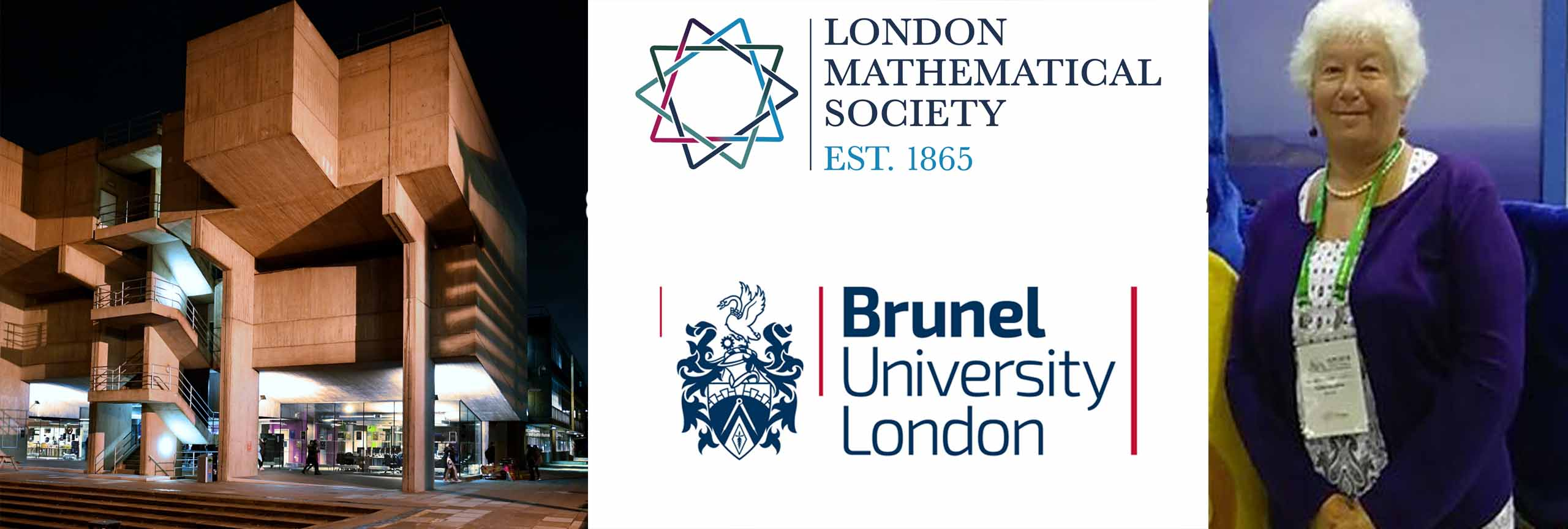# London Mathematical Society Invited Lecture SeriesBrunel University London will be host to the London Mathematical Society Invited Lecture series with Prof Yulia Mishura discussing the connection between Fractional Calculus and Fractional Stochastic Calculus.

Professor Yulia Mishura will give a series of lectures from 30 March to 3 April 2020, on the connection between fractional calculus and fractional stochastic calculus.

The proposed course of lectures is aimed at explaining the mathematical arguments of fractional calculus and fractional stochastic calculus, including rough-path theory, with subsequent application to stochastic differential equations, stochastic partial differential equations, and finance.

The course is aimed at a broad mathematical audience interested in working with long- and short memory processes. In particular it will be beneficial for PhD students. The course will be accompanied by exercise sessions.